## OptSim, OptSim Circuit and ModeSYS: Modeling Stochastic Variations in Parameters and Performance

Accurate modeling of important performance metrics, whether it is for a fiber-optic communication system or a photonic integrated circuit (PIC), depends on the ability to capture inherent randomness associated with underlying physical phenomena, as well as random fluctuations from ambient and production environments. Common examples of stochastic fluctuations include linewidth and population densities affecting light sources and amplifiers, chirp affecting modulation, birefringence and polarization mode dispersion affecting transmission of data through fiber, electrical and optical noise influencing extraction of information (bit error rate), and process variations affecting yield. This article provides an overview of several methods for modeling stochastic behavior performance bounds in OptSim, OptSim Circuit, and ModeSYS.

## OptSim Samplemode

1. Using the Global Seed for the Random Number Generator

Figure 1 shows block diagram of a fiber-optic transmission link and its numerical realization.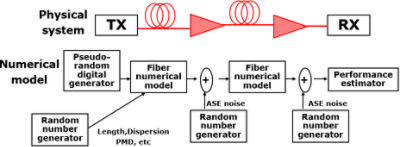Figure 1. A fiber-optic transmission link (top) and its numerical realization (bottom)

In order to simulate all components in a system as shown in Figure 1, many random parameters are generated to obtain the random noise of sources and receivers, and to generate all random parameters for the fiber: length, birefringence, polarization mode dispersion (PMD), and dispersion. OptSim samplemode random generators produce different sets of parameters based on the Random Parameters Seed setting. This setting is defined in the global simulation parameters as shown in Figure 2.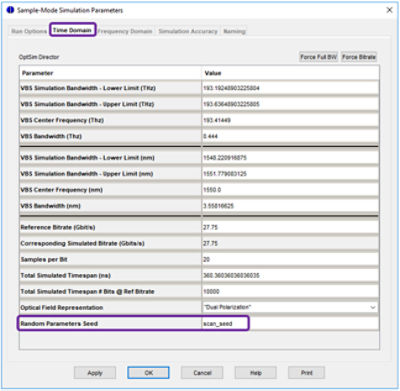Figure 2. Scanning for random parameter seed in samplemode

To re-run the simulation using different random parameters and noises, you must change the seed value. If you perform multiple runs with a varying seed parameter, you can create a Monte Carlo analysis. For example, this procedure may be used to analyze the system impact of PMD in high bit-rate links by automatically re-running the same simulation with different realizations of the PMD stochastic process. Figure 3 depicts Monte Carlo variations in the Q-factor of a link with PMD.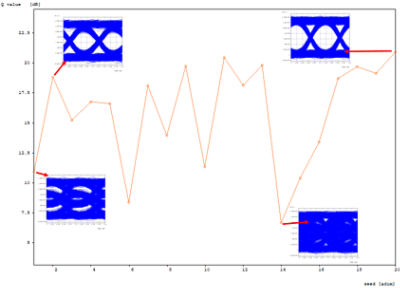Figure 3. Monte Carlo run on fiber PMD and resulting Q-factor

2. Monte Carlo Run on Fiber PMD and Resulting Q-Factor

A component parameter can be assigned a random value with a Gaussian or uniform distribution. To do so, specify a pseudo-random function with the desired statistical parameters in the parameter value field. For instance, you can write in the parameter value field the following expression:

rnd_gauss(Mean, Sigma)

where “Mean” and “Sigma” are defined in the symbol table. In this way, the parameter will assume a random value with Gaussian distribution with the specified Mean and the Sigma values during the simulation:

rnd_unif(min, max)

which returns a pseudo-random value according to a uniform distribution in the range [min,max] where min and max are defined in the symbol table.

The random value is computed at the start of the simulation. If the value is outside the valid range of the parameter, OptSim re-computes it up to 10 times. After ten tries, OptSim will issue an error.

The use of a pseudo-random function in parameter value can also be combined with a scan over random parameter seed as discussed earlier. For example, for a specific value of Mean and Sigma defined in the symbol table, you can set the parameter to the following expression: Mean+rnd_gauss(0.0, Sigma). A scan over global seed will cause this parameter value to assume different Gaussian random variables during each run.

At the end of the simulation, you can see the random values used in each run by clicking Logging on the OptSim menu bar, and then selecting Open Simulation Log File.

On a related note, the pseudo-random data source model has settings to have sequence and polynomial be randomly (or deterministically) selected, and to have the starting point and bit edge randomly (or deterministically) chosen. The latter helps with decorrelation between data channels in multi-channel systems. The all-order PMD model provides a local setting for the seed, which can be scanned for Monte Carlo simulations. Some optical source models allow you to specify deterministic or random
for the starting laser phase.

## OptSim Blockmode, OptSim Circuit, and ModeSYS

1.  Using Pseudo-Random Functions in Parameter Value Definitions

The following functions are available for use in parameter value definitions:

random(), returns a random number between 0.0 and 1.0

gaussian(mean, sigma), returns a random number with Gaussian distribution of specified mean and standard deviation.

An integer argument for random() generates an array of integer number of random numbers, each between 0.0 and 1.0. Other parameters and expressions can use the array elements (random numbers) as follows:

Y = random(4).getElement(2)

or

X = random(4)

Y = X.getElement(2)

to choose the second random number from the array of four random numbers generated by the pseudo-random function.

The samplemode pseudo-random functions described earlier also work in blockmode parameter value definitions. To have these values randomly change during each run, a scan can be created for a variable, say, “x” that is used as an expression involving a pseudo-random function. For example, setting a parameter value to random()+0*x and running a parameter scan over x will cause the parameter value to change randomly in each run.

In transient mode simulations, you can have a parameter assume a different random value during each iteration by specifying the parameter value as an expression involving the pseudo-random function and an internally known variable, currentIteration, that varies during each iteration. An example of such parameter definition is: random()+0*currentIteration

2. Specifying Desired Distribution and Standard Deviation in Parameter Window

As shown in Figure 4, all double-type component parameters provide an option to associate a desired distribution (Uniform, Gaussian, and 2-sigma) with a specified mean and standard deviation.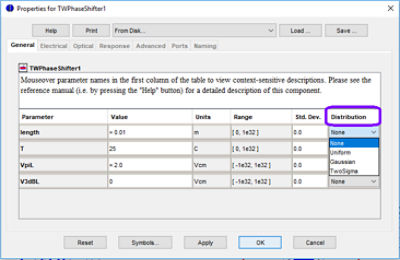Figure 4. Option to choose desired distribution in parameter windows

3. Performing a Statistical Parameter Scan

If you specified any parameter having statistical distribution as mentioned above, you can also perform a parameter scan for a specified number of runs as shown in Figure 5.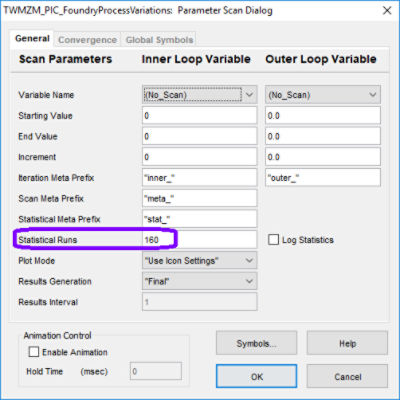Figure 5. Specify desired number of statistical scans in parameter scan setup window

The statistics log (Log Statistics checkbox), if enabled, will be stored for subsequent access. The statistical scan feature is convenient for estimating performance bounds and yield analysis. An example of such an analysis is shown in Figure 6. The plot on the left shows a histogram of the BER spread and the plot on the right shows the distribution of a quantity of interest over a number of random scans.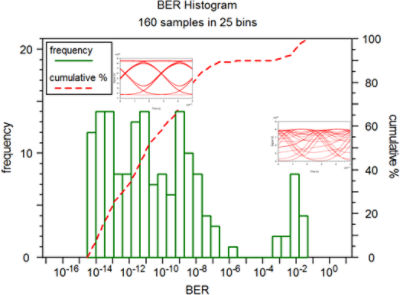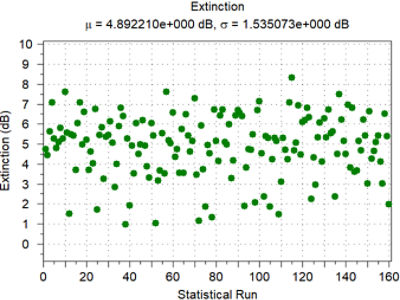Figure 6. Some of the sample results obtained from 160 runs of a statistical parameter scan

On a related note, there are a number of models (such as signal and noise sources, linewidth adder, fiber, receiver, photodetector) that allow you to choose locally the way random numbers are generated for the stochastic quantity used by the model via random seed parameter. The convention is as follows:

• seed < 0

The generator is seeded with the actual value of seed on every run of the simulation. This is useful for obtaining repeatable results.

•  seed = 0

The generator is seeded with an integer hashed from the string value of the component’s name.

• seed = 1

The generator is seeded with a random number obtained from the system clock. This is essentially unrepeatable.

As we can see, there are a number of powerful options available in OptSim, OptSim Circuit, and ModeSYS to perform Monte Carlo analyses and to take into account the random fluctuations of varying time-scale and distribution in model and circuit parameters. For more information, please contact rsoft_support@synopsys.com.

## Calendar of Events

For the latest event calendar, please go to our Events page.